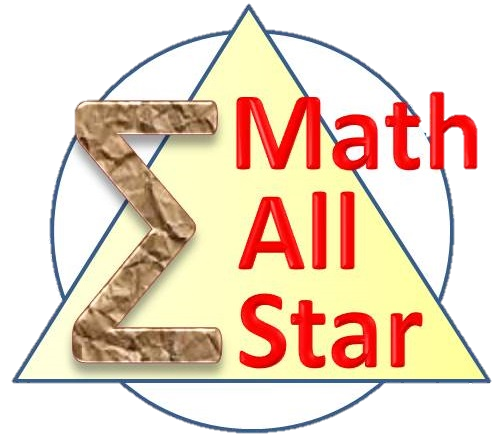#### More on Counting Pre-assessmentProblem - 260
Define an ordered quadruple of integers $(a, b, c, d)$ as interesting if $1 \le a < b < c < d \le 10$, and $a+d>b+c$. How many interesting ordered quadruples are there?
Problem - 4333

A deck of poker has three different colors each of which contains $10$ cards numbered from $1$ to $10$, respectively. In addition, there are two jokers both of which are numbered as $0$. A card with number $k$ is valued as $2^k$ points. How many different ways are there to draw several cards from this deck so that their total value equals $2004$?

Problem - 4355

Let $n$ be a positive integer. Find the number of paths from $(0,0)$ to $(n,n)$ on a $n\times n$ grid using only unit up and right steps so that the path stays in the region $x\ge y$.

Problem - 4356

Find the number of expressions containing $n$ pairs of parentheses which are correctly matched. For example, when $n=3$, the answer is $5$ because all the valid expressions are $$((())),\ (()()),\ (())(),\ ()(()),\ ()()()$$

Problem - 4365

Assuming positive integer $n$ satisfies $n\equiv 1\pmod{4}$ and $n > 1$. Let $\mathbb{P}=\{a_1,\ a_2,\ \cdots,\ a_n\}$ be a permutation of $\{1,\ 2,\ \cdots,\ n\}$. If $k_p$ denotes the largest index $k$ associated with $\mathbb{P}$ such that the following inequality holds $$a_1 + a_2 + \cdots + a_k < a_{k+1}+a_{k+2}+\cdots + a_n$$

Find the sum of $k_p$ for all possible $\mathbb{P}$.

Problem - 4366

How many ways are there to arrange $8$ girls and $25$ boys to sit around a table so that there are at least $2$ boys between any pair of girls? If a sitting plan can be simply rotated to match another one, these two are treated as the same.

Problem - 4407

Dividing a circle into $n \ge 2$ sectors and coloring these sectors using $m\ge 2$ different colors. If no adjacent sectors can be colored the same, how many different color schemes are there?

Problem - 4425

How many $3\times 3$ matrices of non-negative integers are there such that the sum of every row and every column equals $n$?

Problem - 4465

Find the number of ways to divide a convex $n$-sided polygon into $(n-2)$ triangles using non-intersecting diagonals.

Problem - 4478

(Hanoi Tower) There are $3$ identical rods labeled as $A$, $B$, $C$; and $n$ disks of different sizes which can be slide onto any of these three rods. Initially, the $n$ disks are stacked in ascending order of their sizes on $A$. What is the minimal number of moves in order to transfer all the disks to $B$ providing that each move can only transfer one disk to another rod's topmost position and at no time, a bigger disk can be placed on top of a smaller one.